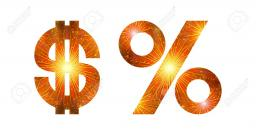# Numbers 5677

The first number is 50% of the second, the second number is 40% of the third, and the third number is 20% of the fourth. The sum is 396. What are the numbers?

a =  12
b =  24
c =  60
d =  300

### Step-by-step explanation:

a= 50/100 b
b = 40/100 c
c = 20/100 d
a+b+c+d = 396

a= 50/100·b
b = 40/100·c
c = 20/100·d
a+b+c+d = 396

100a-50b = 0
100b-40c = 0
100c-20d = 0
a+b+c+d = 396

Row 4 - 1/100 · Row 1 → Row 4
100a-50b = 0
100b-40c = 0
100c-20d = 0
1.5b+c+d = 396

Row 4 - 1.5/100 · Row 2 → Row 4
100a-50b = 0
100b-40c = 0
100c-20d = 0
1.6c+d = 396

Row 4 - 1.6/100 · Row 3 → Row 4
100a-50b = 0
100b-40c = 0
100c-20d = 0
1.32d = 396

d = 396/1.32 = 300
c = 0+20d/100 = 0+20 · 300/100 = 60
b = 0+40c/100 = 0+40 · 60/100 = 24
a = 0+50b/100 = 0+50 · 24/100 = 12

a = 12
b = 24
c = 60
d = 300

Our linear equations calculator calculates it.Did you find an error or inaccuracy? Feel free to write us. Thank you!

Tips for related online calculators